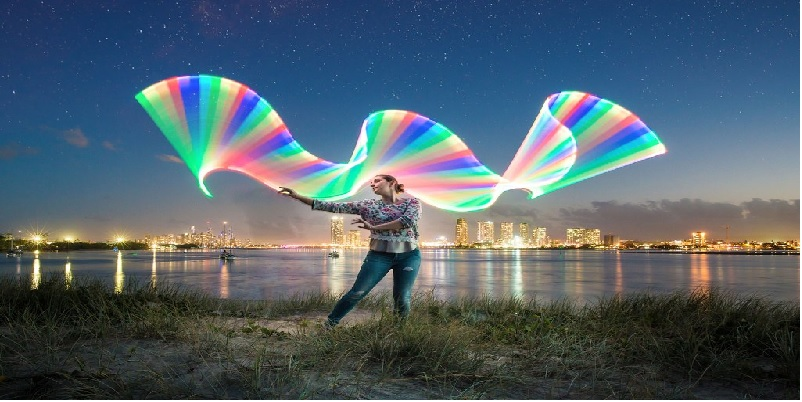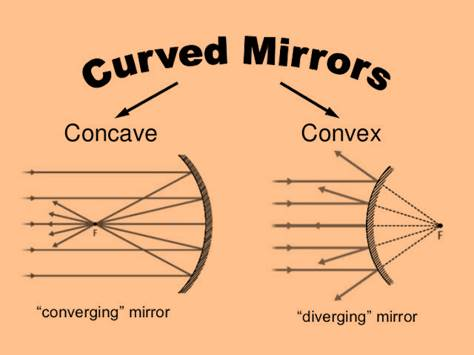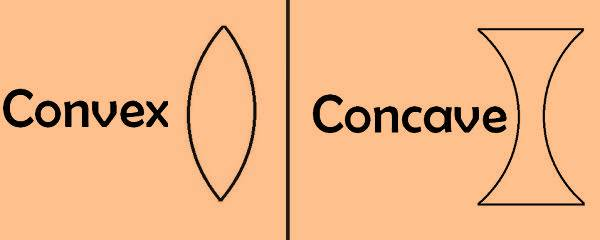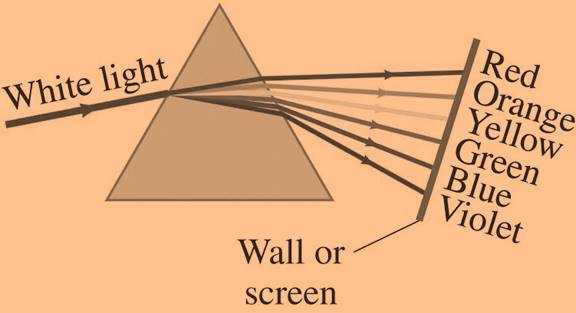Register for Maths, Science, English, Reasoning Olympiad Exams Register here | Book Free Demo for Live Olympiad Classes here| Check Olympiad Exam Dates here | Buy Practice Papers for IMO, IOM, HEO, IOEL etc here | Login here to participate in all India free mock test on every Saturday

# Characteristics Of Light• Light is never curved and the path is always straight.
• Only reflection can change the direction of light.
• When light bounce by smooth surface then it is called as reflection of light.

## Image

• When the impression of an object created in a mirror due to the reflection by light then it is known as the image of the object.
• A distance of the image increases with the distance increase in the object.

### Characteristics of an image

1. The image is left-right inversed
2. The size is same like object.
3. Virtual and erect

### Spherical mirror

• Also called as curved mirrors due to having curved reflecting surfaces.
• At each hemisphere, two types of the curved surface occur
• Concave mirror and convex mirror are the two type of spherical mirror.

### Concave mirror

• A concave mirror is one which has a concave reflecting surface.
• Also known as converging mirror.
• A dentist uses concave mirrors.
• Also used in headlights of scooters and cars

### Convex mirror

• A convex mirror is one which has a convex reflecting surface.
• Also called as fish eye mirror or diverging mirror
• The image formed is virtual, diminished and erect.
• As a security measure used in supermarkets, shops and ATM centers.### Opaque spherical surface

• The silvered surface in an opaque spherical surface acts as a mirror.
• A convex mirror will have convex side silvered and concave will have concave side silvered

### Concave lens

• When at least one surface of a lens is curved inwards then it is known as a concave lens.
• The rays are divergent.
• The centre is thinner than the edges.
• The image formed is virtual and upright.

### Convex lens

• An object becomes magnified.
• Light rays are converged.
• The image formed is erect, magnified and virtual.### Application of lenses

• Peepholes
• Magnifying glasses
• Bioscopes
• Cameras
• Telescopes
• Binoculars
• Projectors
• Microscopes

### Dispersion of light through a prism

• Have a glass prism
• Then let the beam of sunlight to go through the small hole in the window to fall on one side of a prism
• The light when passing through prism bends.
• Now let the light go out from another side of the prism to fall on white paper.
• The colors will disperse.## Recap

• Light is never curved and the path is always straight.
• Only reflection can change the direction of light.
• The distance of the image increases with the distance increase in the object.
• The image is left-right inversed
• A concave mirror is one which has a concave reflecting surface.
• A convex mirror is one which has a convex reflecting surface.